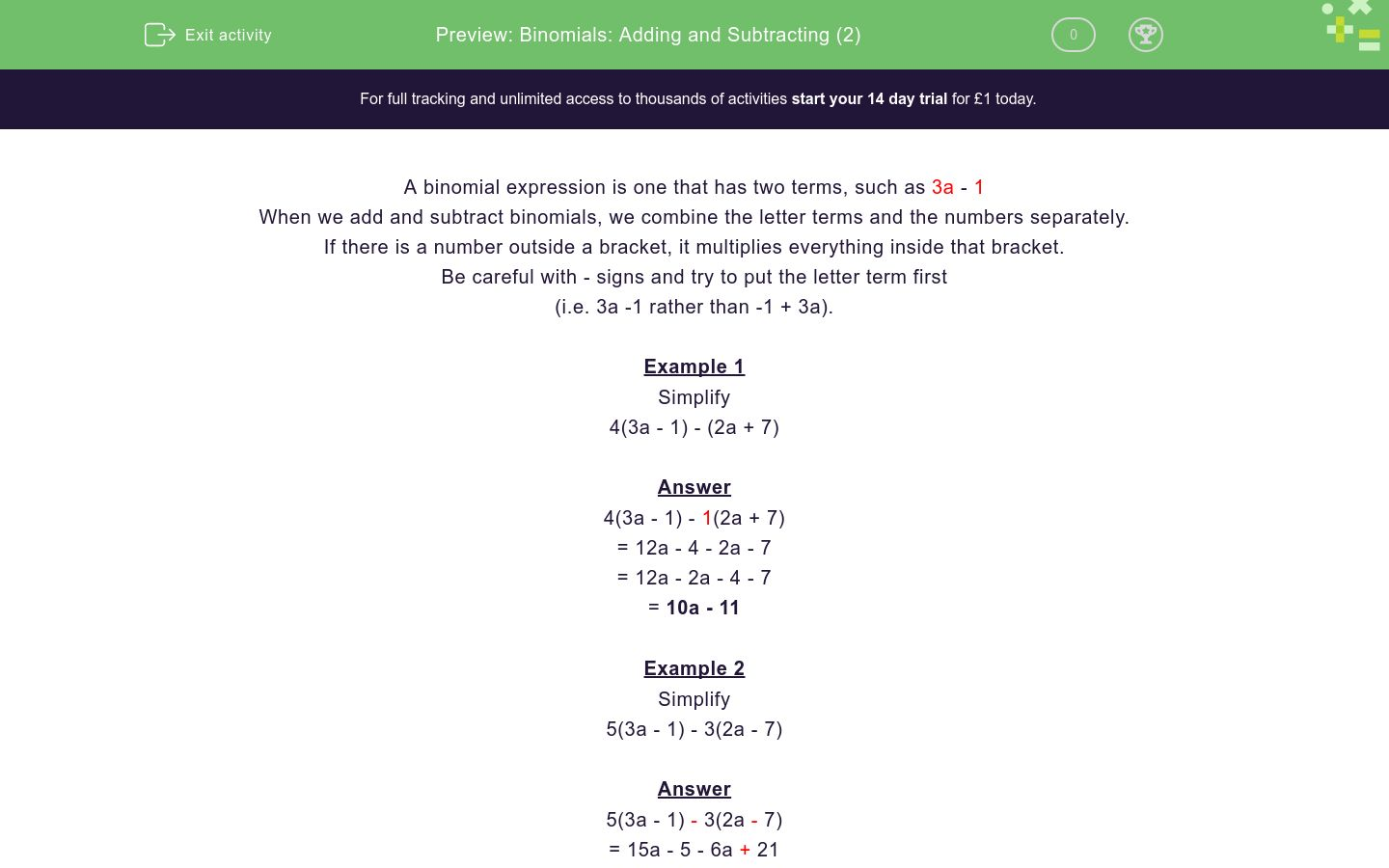# Binomials: Adding and Subtracting (2)

In this worksheet, students add and subtract binomial expressions.Key stage:  KS 4

Curriculum topic:  Algebra

Difficulty level:### QUESTION 1 of 10

A binomial expression is one that has two terms, such as 3a - 1

When we add and subtract binomials, we combine the letter terms and the numbers separately.

If there is a number outside a bracket, it multiplies everything inside that bracket.

Be careful with - signs and try to put the letter term first

(i.e. 3a -1 rather than -1 + 3a).

Example 1

Simplify

4(3a - 1) - (2a + 7)

4(3a - 1) - 1(2a + 7)

= 12a - 4 - 2a - 7

= 12a - 2a - 4 - 7

= 10a - 11

Example 2

Simplify

5(3a - 1) - 3(2a - 7)

5(3a - 1) - 3(2a - 7)

= 15a - 5 - 6a + 21

= 15a - 6a - 5 + 21

= 9a + 16

Example 3

Simplify

6(3a + 1) - 3(2 - 7a)

6(3a + 1) - 3(2 - 7a)

= 18a + 6 - 6 + 21a

= 18a + 21a + 6 - 6

= 39a

Simplify

3(4a - 2) + (2a + 5)

Simplify

(13a - 2) - 2(2a + 5)

Simplify

2(13a - 2) - (12a - 5)

Simplify

2(15a - 15) + 2(15a + 15)

Simplify

2(5a + 12) + (15a - 12)

Simplify

3(5a + 1) - 2(5a - 1)

Simplify

3(5a + 1) - 2(-5a + 1)

Simplify

6(3a + 1) - 3(6 + a)

Simplify

4(3a + 1) - 2(3 - a)

Simplify

4(-3a + 1) - 5(3 - a)

• Question 1

Simplify

3(4a - 2) + (2a + 5)

14a-1
• Question 2

Simplify

(13a - 2) - 2(2a + 5)

9a-12
• Question 3

Simplify

2(13a - 2) - (12a - 5)

14a+1
• Question 4

Simplify

2(15a - 15) + 2(15a + 15)

60a
• Question 5

Simplify

2(5a + 12) + (15a - 12)

25a+12
• Question 6

Simplify

3(5a + 1) - 2(5a - 1)

5a+5
• Question 7

Simplify

3(5a + 1) - 2(-5a + 1)

25a+1
• Question 8

Simplify

6(3a + 1) - 3(6 + a)

15a-12
• Question 9

Simplify

4(3a + 1) - 2(3 - a)

14a-2
• Question 10

Simplify

4(-3a + 1) - 5(3 - a)